« Example 2 Clarifications » Example 3 (project <Β_422-3>) Region of three-edge- supported slabs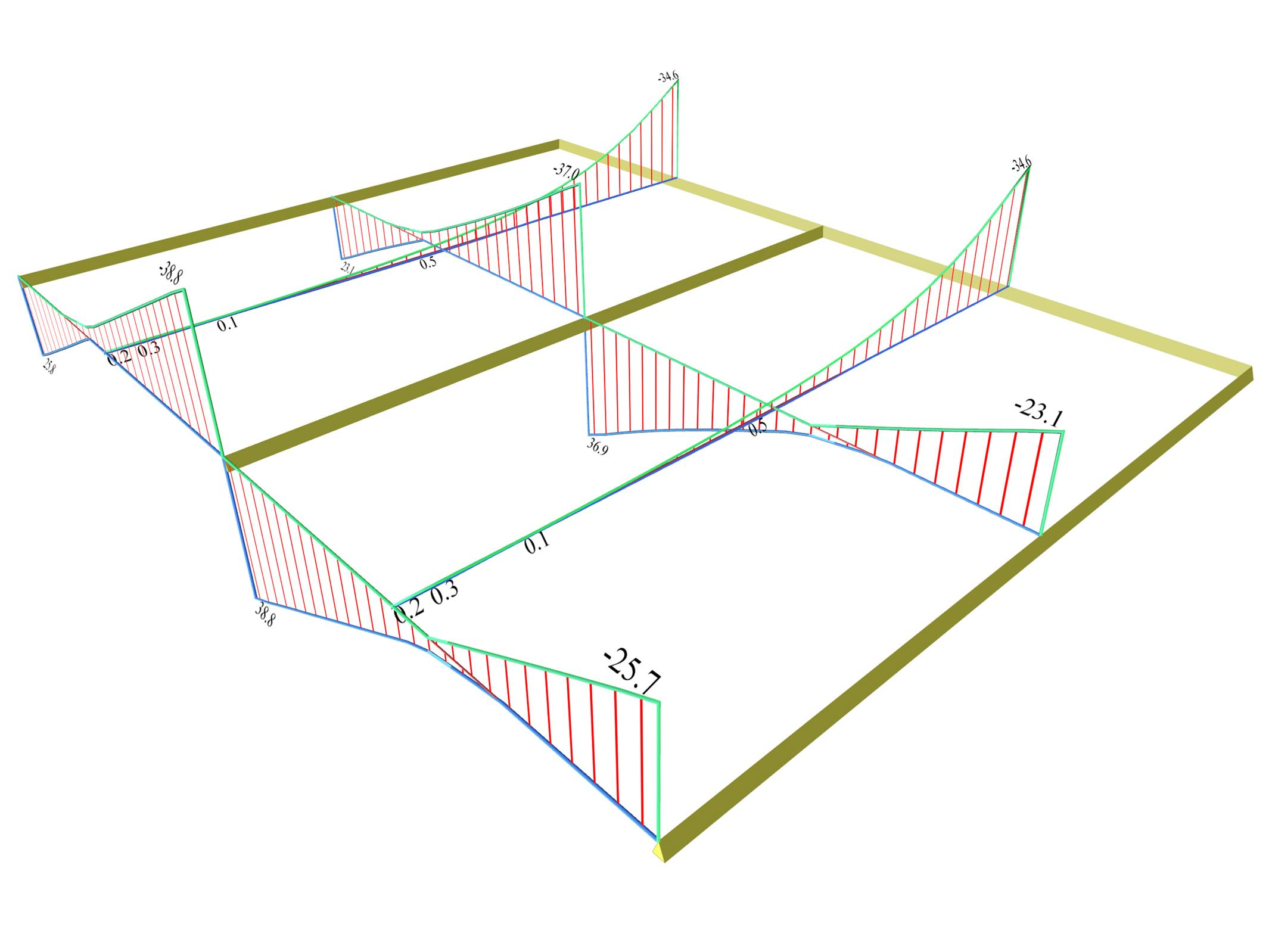Figure 4.2.3-21: Shear force envelopes Figure 4.2.3-21: Shear force envelopes The most unfavourable shear forces are equal to: Middle cross-section in x: Vxr=23.1 (21.1) [*]NoteThe respective results corresponding to global loading are shown in brackets. , Vx,erm=-37.0 (-36.4) [kN] End cross-section in x: Vxr=25.8 (23.1), Vx,erm=-38.8 (-38.3) [kN] y direction: Vy,erm=-34.6 (-31.3) [kN]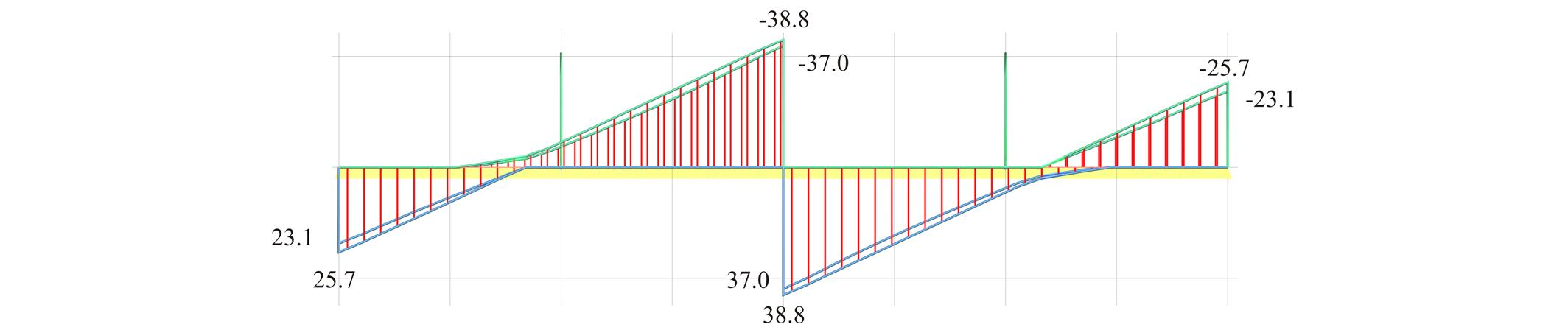Figure 4.2.3-22: Front view of the 3D shear force diagrams, corresponding to the envelope of [Vx] Figure 4.2.3-22: Front view of the 3D shear force diagrams, corresponding to the envelope of [Vx]Figure 4.2.3-23: Side view of the 3D shear force diagrams, corresponding to the envelope of [Vy] Figure 4.2.3-23: Side view of the 3D shear force diagrams, corresponding to the envelope of [Vy] Note that although the live load has a relatively high value, the differences between the shear forces do not exceed 9%.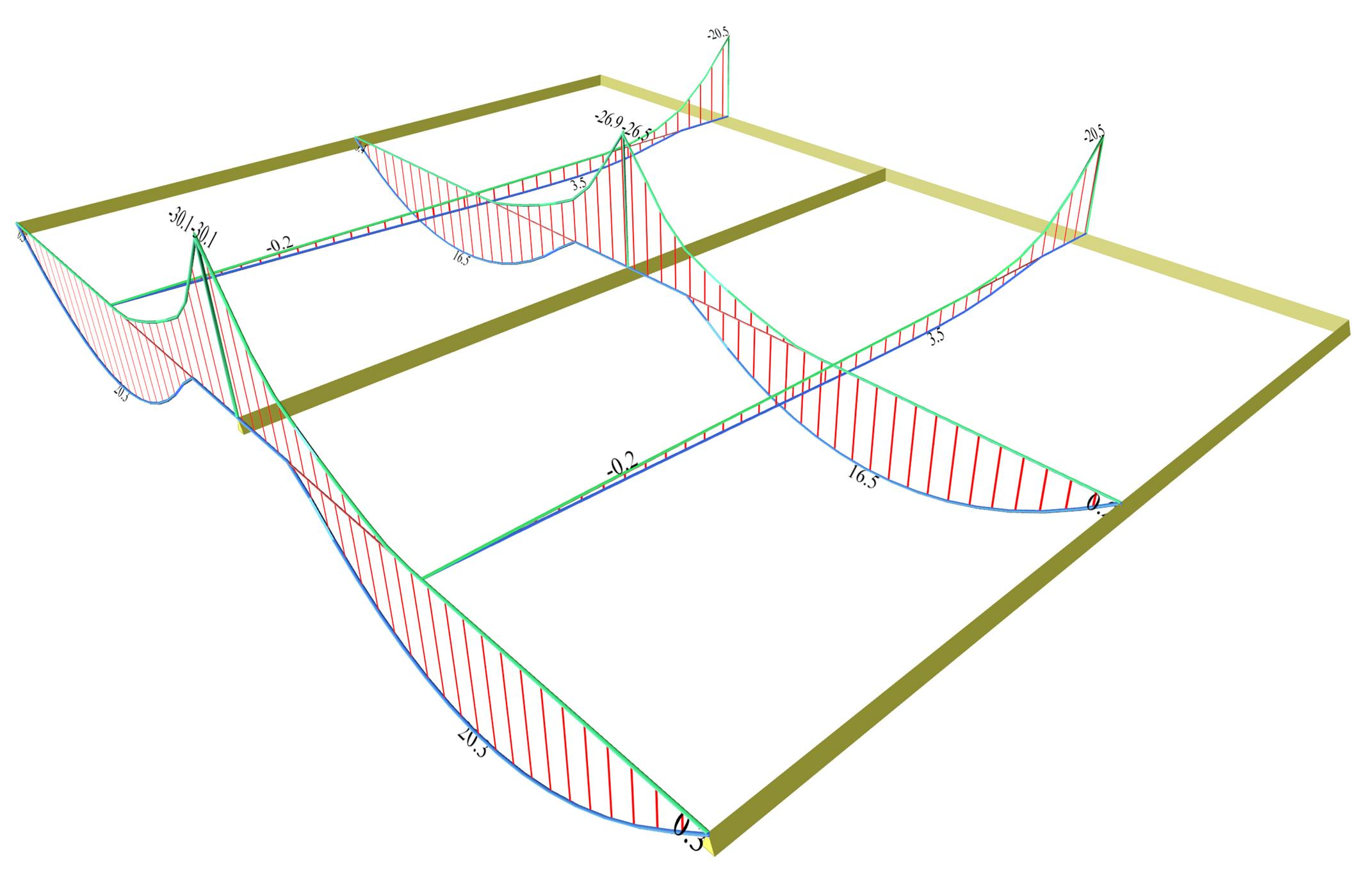Figure 4.2.3-24: Bending moment envelopes Figure 4.2.3-24: Bending moment envelopes The most unfavourable bending moments are equal to: Middle cross-section in x: Μx=16.5 (13.8) [*]NoteThe respective results corresponding to global loading are shown in brackets. , Mx,erm=-26.7 (-26.6) [kNm] End cross-section in x: Mx=20.3 (16.9), Mx,erm=-29.9 (-29.9) [kNm] y direction: My=3.5 (2.8), My,erm=-20.5 (-17.4) [kNm]Figure 4.2.3-25: Front view of the 3D bending moment diagrams, corresponding to the envelope of [Mx] Figure 4.2.3-25: Front view of the 3D bending moment diagrams, corresponding to the envelope of [Mx](Fig. 4.2.3_26) Side view of the 3D bending moment diagrams, corresponding to the envelope of [My] (Fig. 4.2.3_26) Side view of the 3D bending moment diagrams, corresponding to the envelope of [My] Notice that although the live load has a relatively high value, the differences between the bending moments are small.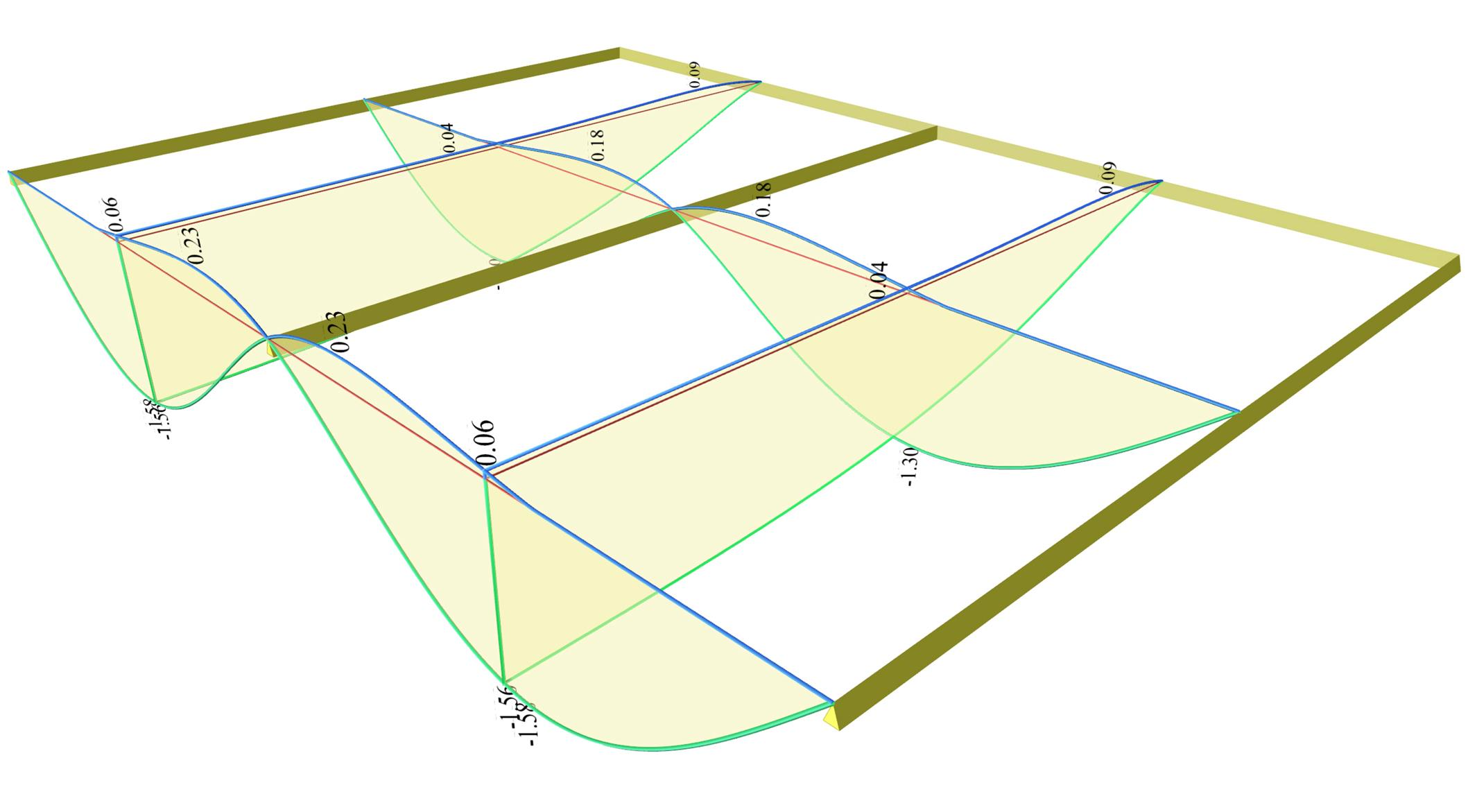Figure 4.2.3-27: Deflection envelopes Figure 4.2.3-27: Deflection envelopes Maximum deflections of y=-1.58 (-1.14 ) [*]NoteThe respective results corresponding to global loading are shown in brackets. mm occur at the free edges of the three-edge-supported slabs. At the same edges opposite sign deformation of y=0.23 mm is also developed. In the middle cross-section of the slabs the extreme deflections are y=-1.30(- 0.94) mm and y=0.18 mm respectively.Figure 4.2.3-28: Front view of the 3D deflection diagrams Figure 4.2.3-28: Front view of the 3D deflection diagramsFigure 4.2.3-29: Side view of the 3D deflection diagrams Figure 4.2.3-29: Side view of the 3D deflection diagrams Note that the deflections are quite higher reaching up to 40% and, at the same time, opposite sign deformations also arise (blue lines). Cantilever region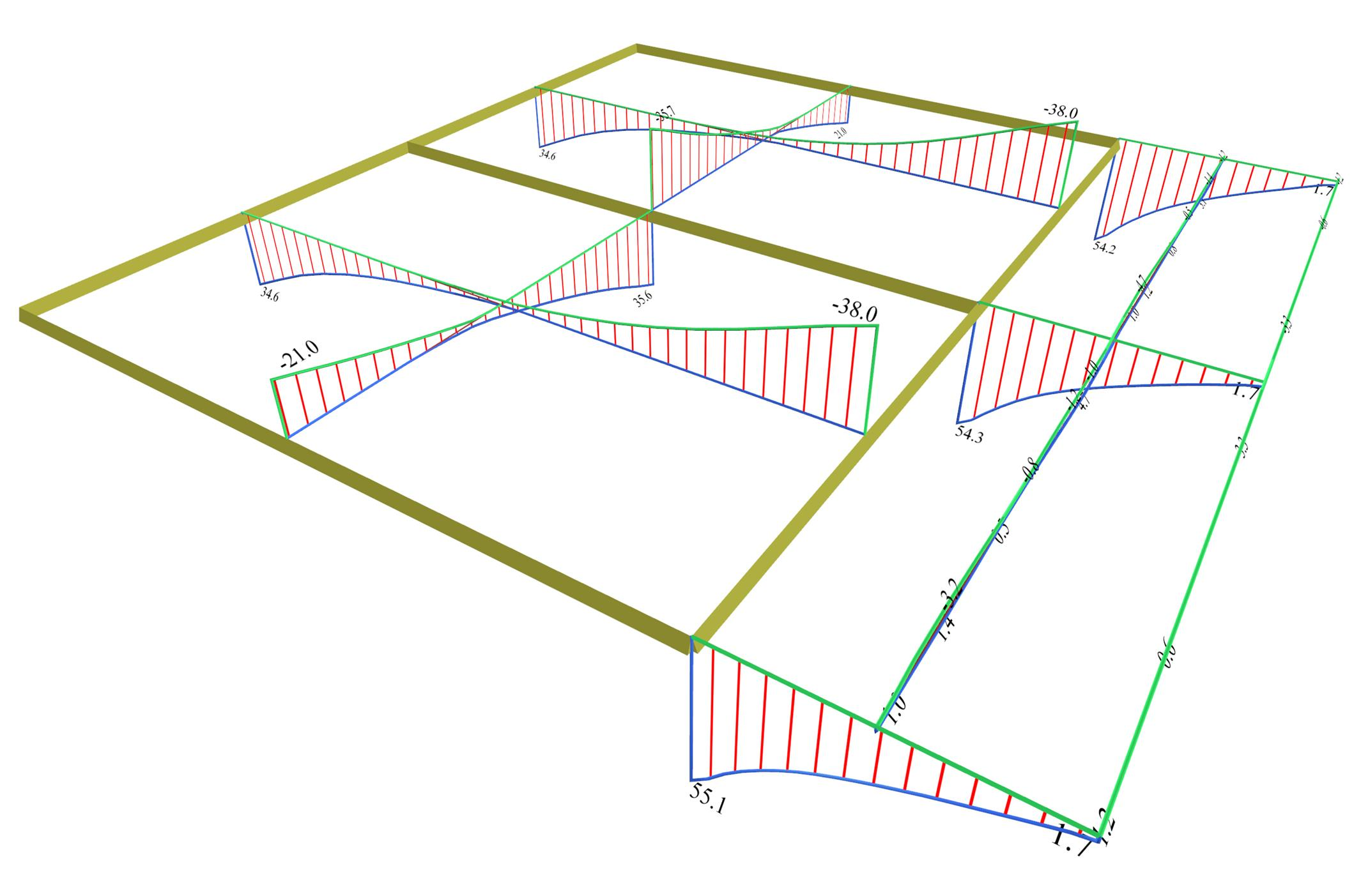Figure 4.2.3-30: Shear force envelopes Figure 4.2.3-30: Shear force envelopes The most unfavourable shear forces are equal to: Two-way in x: Vxr=20.0 (18.6) [*]NoteThe respective results corresponding to global loading are shown in brackets. , Vx,erm=-35.7 (-33.6) [kN] Cantilever in x: small shear forces Two-way in y: Vyr=34.6 (31.4), Vy,erm=-38.0 (-35.9) [kN] Cantilever in y: Vy,erm=54.3 (46.9) [kN]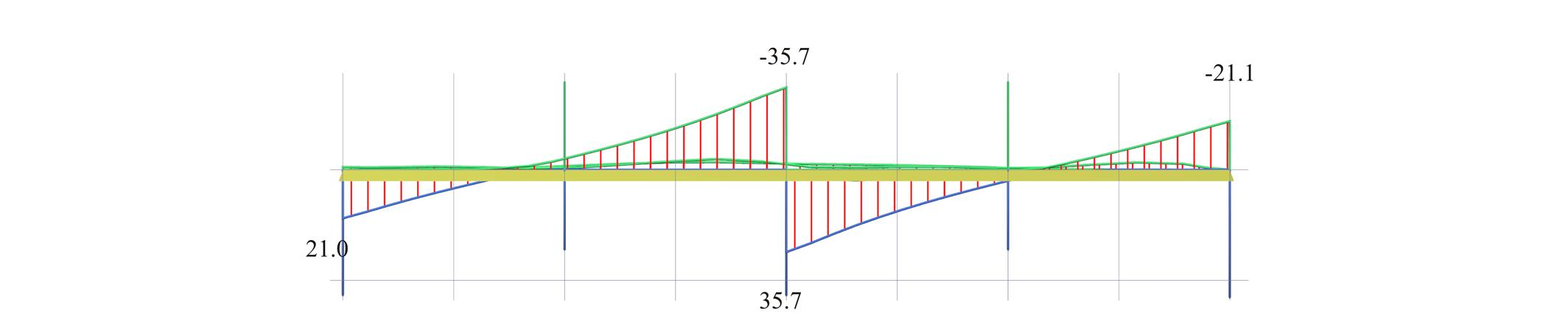Figure 4.2.3-31: Front view of the 3D shear force diagrams, corresponding to the envelope of [Vx] Figure 4.2.3-31: Front view of the 3D shear force diagrams, corresponding to the envelope of [Vx]Figure 4.2.3-32: Side view of the 3D shear force diagrams, corresponding to the envelope of [Vy] Figure 4.2.3-32: Side view of the 3D shear force diagrams, corresponding to the envelope of [Vy] Notice that although the live load has a relatively high value, the differences between the shear forces do not exceed 6% except for the peak of the cantilever middle support reaching 15%.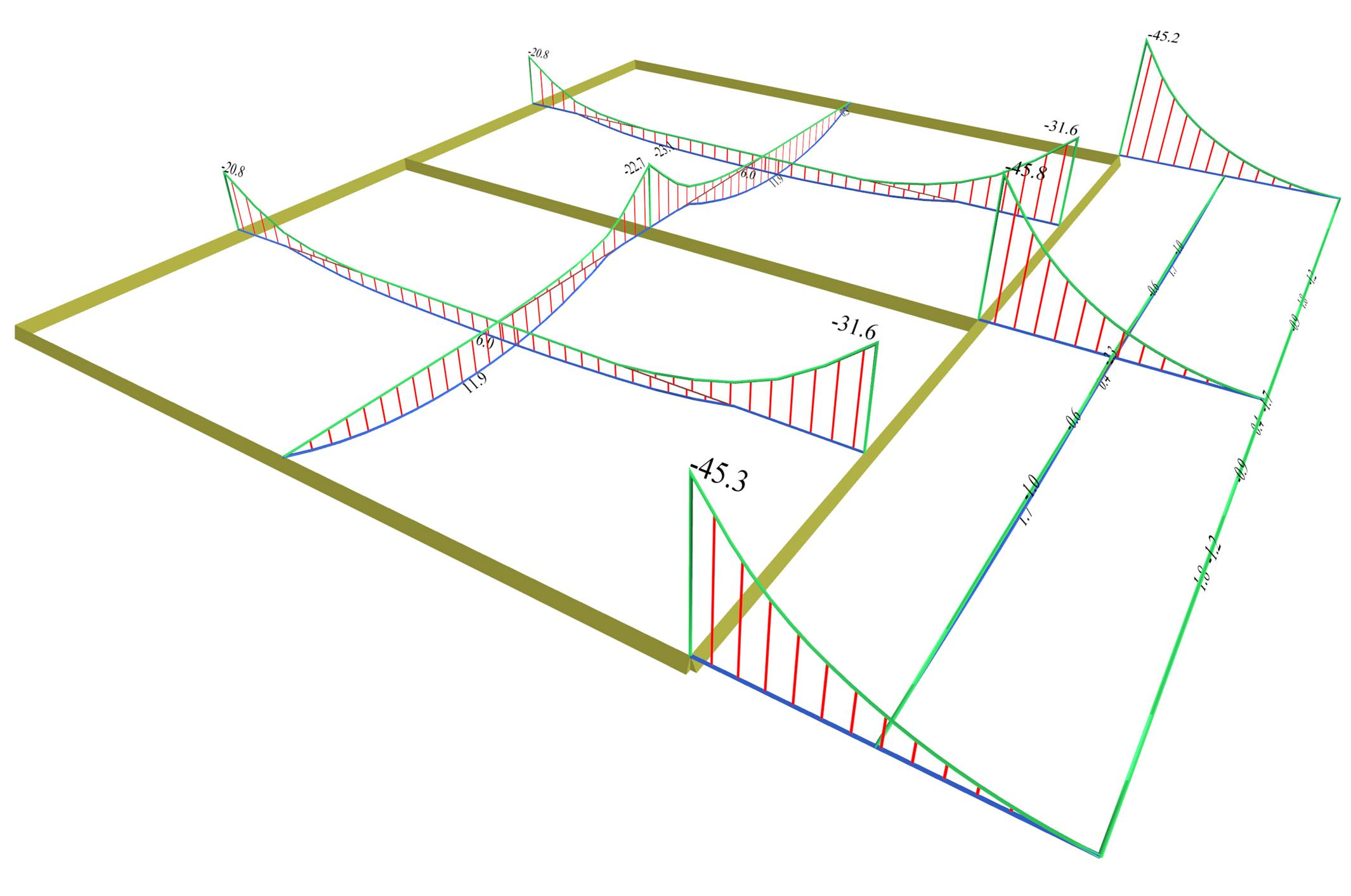Figure 4.2.3-33: Bending moment envelopes Figure 4.2.3-33: Bending moment envelopes The most unfavourable bending moments are equal to: Two-way in x: Μx=11.9 (8.0) [*]NoteThe respective results corresponding to global loading are shown in brackets. , Mx,erm=-22.8 (-19.9) [kNm] Cantilever in x: small moments Two-way in y: Μy=6.0 (4.9), My,erm,1=-20.5 (-17.4) and My,erm,2=-31.6 (-30.6) [kNm] Cantilever in y: extended My,erm =-31.6 (-30.6), peak -45.8 (-41.3) [kNm]Figure 4.2.3-34: Front view of the 3D bending moment diagrams, corresponding to the envelope of [Mx] Figure 4.2.3-34: Front view of the 3D bending moment diagrams, corresponding to the envelope of [Mx]Figure 4.2.3-35: Side view of the 3D bending moment diagrams, corresponding to the envelope of [My] Figure 4.2.3-35: Side view of the 3D bending moment diagrams, corresponding to the envelope of [My] The differences between the unfavourable bending moments and the bending moments due to global loading, range from 15 to 50%.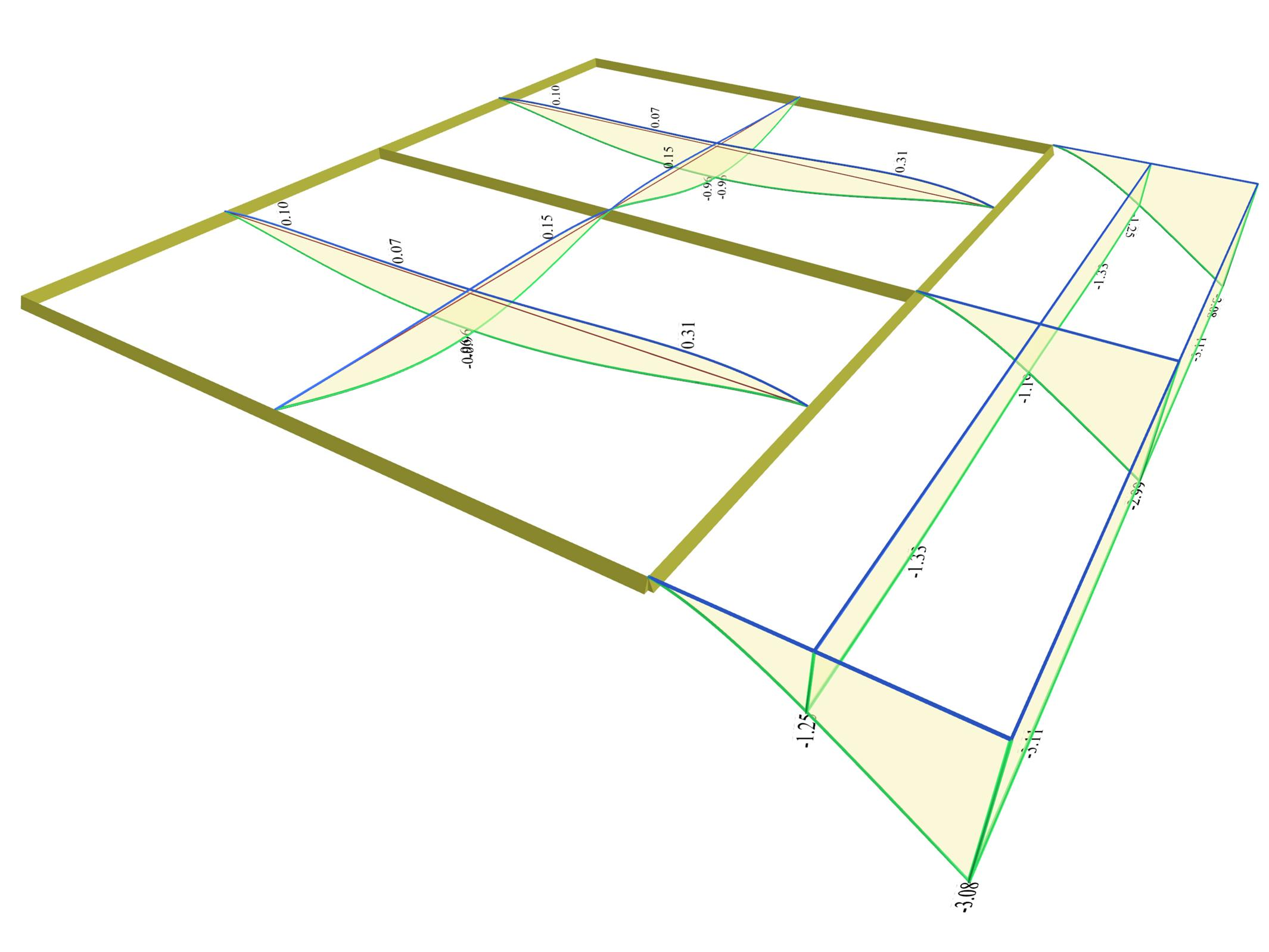Figure 4.2.3-36: Deflection envelopes Figure 4.2.3-36: Deflection envelopes Maximum deflection of y=-0.96 (-0.64) [*]NoteThe respective results corresponding to global loading are shown in brackets. mm and opposite sign deflection of y=0.31 (0.09) mm occur in two-way slabs. In cantilever slab the maximum deflection is y=-3.11 (-2.81) mm.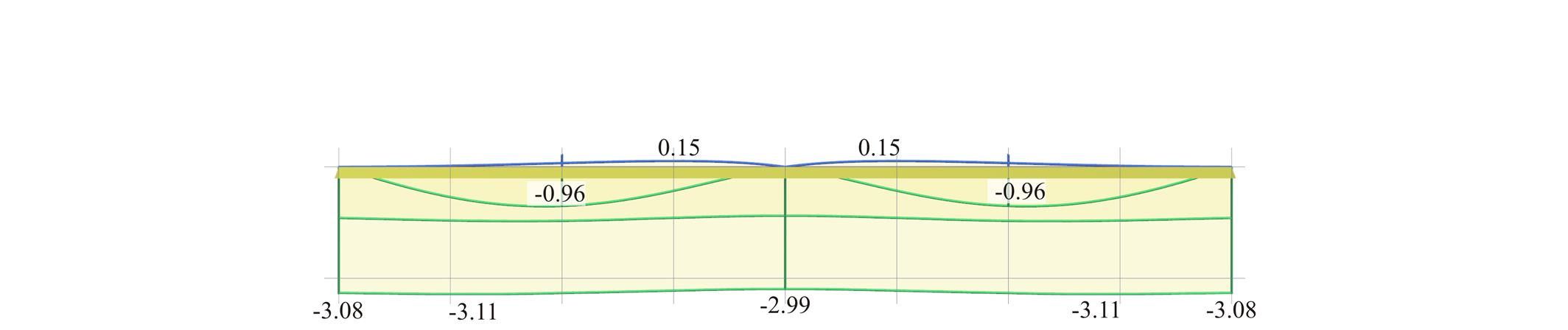Figure 4.2.3-37: Front view of the 3D deflection diagrams Figure 4.2.3-37: Front view of the 3D deflection diagramsFigure 4.2.3-38: Side view of the 3D deflection diagrams Figure 4.2.3-38: Side view of the 3D deflection diagrams The slightly fluctuating deformations of the cantilever longitudinal free edge, are due to the fluctuations of the two-way slabs deformations behind the cantilever. « Example 2 Clarifications »